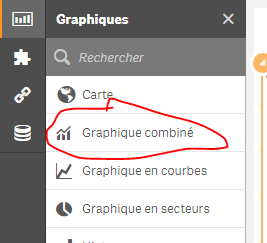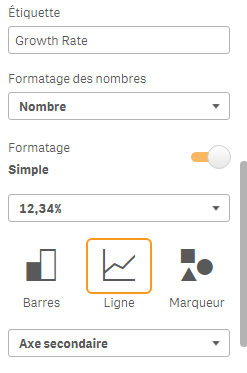# New to Qlik Sense

Discussion board where members can get started with Qlik Sense.

Announcements
Coming your way, the Qlik Data Revolution Virtual Summit. October 27-29. REGISTER
cancel
Showing results for
Did you mean:
HighlightedNot applicable

## Growth Rate

I need an expression that returns de growth rate in QLIK SENSE  combo chart. the expression must be connected to the Date and sales table.

I´m going to show an example:1 Solution

Accepted Solutions
HighlightedNot applicable

thanks to both of you

9 Replies
HighlightedMVP

May be like this

Sum(Sales)/Above(Sum(Sales)) - 1

HighlightedNot applicable

No.

I have to use an expression that return the dates in date column to years (bar chart) and secondary axis is a line with an expression like the example in excel i have shown for growth rate.

HighlightedPartner

It's exactly what stalwar1‌ proposed:

You should use a combined graphas dimension : Year

As measures:

1) Sum({<Year=>}sales)

2) (Sum({<Year=>}[sales])-above(Sum({<Year=>}[sales])))

/

above(Sum({<Year=>}[sales])) : choose representation Line and it must be a secondary axis (format it as %):Result:HighlightedNot applicable

thanks to both of you

HighlightedMVP

What is the point of marking a thank you response as a correct answer? Might as well leave it open....

HighlightedNot applicable

I used the same expression to build a KPI showing the growth rate between 2015-2017, but the result was wrong.

(Sum({<Year={'2015'}>}[sales])-above(Sum({<Year={'2017'}>}[sales])))/above(Sum({<Year={'2017'}>}[sales]))

Can you help me?

HighlightedPartner

You should proceed as this:

1) measure 1 :  Sum({<Year={"<=2017 >=2015"}>}sales)

2) (Sum({<Year={"<=2017 >=2015"}>}[sales])-above(Sum({<Year={"<=2017 >=2015"}>}[sales])))

/

above(Sum({<Year={"<=2017 >=2015"}>}[sales]))

HighlightedPartner

I thought you were using the graphic above and want to only see the years 2015 to 2017

Here's the expression if you want to compare 2017 % 2015:

(Sum({<Year={'2017'}>}sales)-Sum({<Year={'2015'}>}sales))

/

Sum({<Year={'2015'}>}sales)

HighlightedContributor

Hello,

is there a way to have a graph like this but with the possibility to select the years?

because with <Year=> user selection doesn't work and all the years are shown.

Thank you